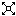### Unit 4 - The Simplex Algorithm - Part 2

ID: 59764
Creator: RUIZ FEMENIA, PEDRO DAVIDCategory: GeologyClasification Unesco: Mathematics::Operations research::Linear programming
Description: Theory Lecture: Unit 4 of Mathematics II course of ADE - The simplex algorithm (part 2). Power point presentation with audio narration.
Labels:
Resolution:640 x 4804:3
Score: Sense puntuacio (puntuar).
License CC: Reconocimiento - NoComercial (by-nc)a
Visits: 6

##### related videos#### Exercise 9 - Unit 4

Solution to theoretical exercise 9 of Unit 4 - The Simplex Algorithm. Course Mathematics II of ADE, group ARA. Power point slides with audio narration.#### Exercises 1, 4 and 8 - Unit 4

Solution to theoretical exercises 1, 4 and 8 of Unit 4 - The Simplex Algorithm. Course Mathematics II of ADE, group ARA. Power point slides with audio narration.#### Unit 5 - Integer Linear Programming

Theory Lecture: Unit 6 of Mathematics II course of ADE - Unit 6 - Integer Linear Programming.#### Unit 3 - Introduction to Linear Programming

Theory Lecture: Unit 3 of Mathematics II course of ADE - An Introduction to Linear Programming. Power point presentation with audio narration.#### Unit 4 - The Simplex Algorithm

Theory Lecture: Unit 4 of Mathematics II course of ADE - The simplex algorithm (part 1). Power point presentation with audio narration.